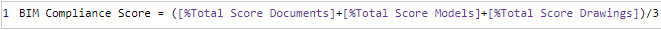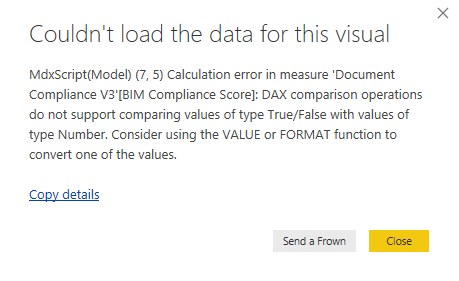cancel
Showing results for
Did you mean:Helper I

## Average of multiple measures

Currently I use 3 measures to calculate an average percentage using a (x+y+z)/3 method.The problem I have however is that there will be no values for one of the measures in some instances but the total will still be divided by three.

Is there a formula that I can use to calculate the average of the three measures whilst ignoring any measures that contain no values?

1 ACCEPTED SOLUTIONSuper User

It's the comparisons that yield the booleans . Cast them to int:

```Measure =
VAR Count_ =
INT ( [Measure1] <> BLANK () )
+ INT ( [Measure2] <> BLANK () )
+ INT ( [Measure3] <> BLANK () )
RETURN
DIVIDE ( [Measure1] + [Measure2] + [Measure3], Count_ )
```

or otherwise:

```Measure =
VAR Count_ =
IF ( [Measure1] <> BLANK (), 1, 0 )
+ IF ( [Measure2] <> BLANK (), 1, 0 )
+ IF ( [Measure3] <> BLANK (), 1, 0 )
RETURN
DIVIDE ( [Measure1] + [Measure2] + [Measure3], Count_ )```

10 REPLIES 10New Member

Thanks for your help. This saved me a ton of time.Super User

Something like

```Measure =
VAR Count_ =
[Measure1] <> BLANK () + [Measure2] <> BLANK () + [Measure3] <> BLANK ()
// Check how many are non-blank
RETURN
DIVIDE ( [Measure1] + [Measure2] + [Measure3], Count_ ) // And divide by that count
```Helper I

Thank you for your response @AlB

I have inputed the suggested code however now, when updating the visuals, I recieve the following error:Do you have any ideas?

No values in any of the measures or related values are categorised as TRUE/FALSE so I'm lost!Super User

It's the comparisons that yield the booleans . Cast them to int:

```Measure =
VAR Count_ =
INT ( [Measure1] <> BLANK () )
+ INT ( [Measure2] <> BLANK () )
+ INT ( [Measure3] <> BLANK () )
RETURN
DIVIDE ( [Measure1] + [Measure2] + [Measure3], Count_ )
```

or otherwise:

```Measure =
VAR Count_ =
IF ( [Measure1] <> BLANK (), 1, 0 )
+ IF ( [Measure2] <> BLANK (), 1, 0 )
+ IF ( [Measure3] <> BLANK (), 1, 0 )
RETURN
DIVIDE ( [Measure1] + [Measure2] + [Measure3], Count_ )```Regular Visitor

You saved my day! THANKS A LOT!New Member

Awesome, Thanks a ton for this!New Member

Thank you for this solution it is exactly what I was looking for...New Member

Hey, this works great.

``````Measure =
VAR Count_ =
INT ( [Measure1] <> BLANK () )
+ INT ( [Measure2] <> BLANK () )
+ INT ( [Measure3] <> BLANK () )
RETURN
DIVIDE ( [Measure1] + [Measure2] + [Measure3], Count_ )``````

But if i want to add the ALL function to this, ignoring filtering - how would that be done?Super User

Hi  Something like

``CALCULATE([Measure1], ALL (Table1) )``

applied to each of the measures should work. You would need to provide more details for a more accurate answer though

Please mark the question solved when done and consider giving kudos if posts are helpful.

CheersHelper I

Works a charm.

- Cameron

@AlB wrote:

It's the comparisons that yield the booleans . Cast them to int:

```Measure =
VAR Count_ =
INT ( [Measure1] <> BLANK () )
+ INT ( [Measure2] <> BLANK () )
+ INT ( [Measure3] <> BLANK () )
RETURN
DIVIDE ( [Measure1] + [Measure2] + [Measure3], Count_ )```

or otherwise:

```Measure =
VAR Count_ =
IF ( [Measure1] <> BLANK (), 1, 0 )
+ IF ( [Measure2] <> BLANK (), 1, 0 )
+ IF ( [Measure3] <> BLANK (), 1, 0 )
RETURN
DIVIDE ( [Measure1] + [Measure2] + [Measure3], Count_ )```